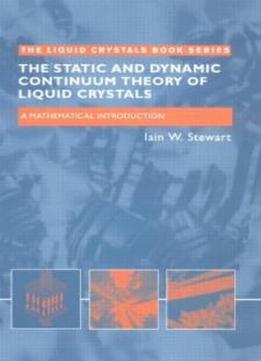The Static And Dynamic Continuum Theory Of Liquid Crystals: A Mathematical Introduction (liquid Crystals Book Series) by Iain W. Stewart / 2004 / English / DjVu

Given the widespread interest in macroscopic phenomena in liquid crystals, stemming from their applications in displays and devices. The need has arisen for a rigorous yet accessible text suitable for graduate students, whatever their scientific background. This book satisfies that need.

Given the widespread interest in macroscopic phenomena in liquid crystals, stemming from their applications in displays and devices. The need has arisen for a rigorous yet accessible text suitable for graduate students, whatever their scientific background. This book satisfies that need. The approach taken in this text, is to introduce the basic continuum theory for nematic liquid crystals in equilibria, then it proceeds to simple application of this theory- in particular, there is a discussion of electrical and magnetic field effects which give rise to Freedericksz transitions, which are important in devices. This is followed by an account of dynamic theory and elementary viscometry of nemantics Discussions of backflow and flow-induced instabilities are also included. Smetic theory is also briefly introduced and summarised with some examples of equilibrium solutions as well as those with dynamic effects. A number of mathematical techniques, such as Cartesian tensors and some variational calculus, are presented in the appendices.

The approach taken in this text, is to introduce the basic continuum theory for nematic liquid crystals in equilibria, then it proceeds to simple application of this theory- in particular, there is a discussion of electrical and magnetic field effects which give rise to Freedericksz transitions, which are important in devices. This is followed by an account of dynamic theory and elementary viscometry of nemantics Discussions of backflow and flow-induced instabilities are also included. Smetic theory is also briefly introduced and summarised with some examples of equilibrium solutions as well as those with dynamic effects. A number of mathematical techniques, such as Cartesian tensors and some variational calculus, are presented in the appendices.

views: 537